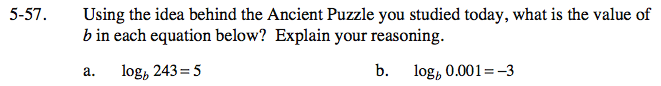5-57.

### Missing problem for Book int3s => Chapter Ch5 => Lesson 5.2.1 => Problem 5-57

Created from orphaned homework help problem$b^5 = 243$

$0.001=\frac{1}{10^3}$